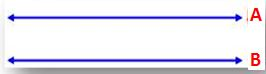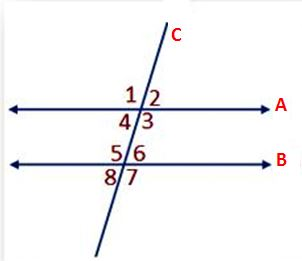# Properties of Parallel Lines -Definition, Theorem & Examples

Parallel Lines are two lines in a plane, they do not intersect and parallel to each other. The distance between the parallel lines is always the same. The symbol to denote parallel lines is ||. Learn and practice all Lines and Angles concepts and problems on our website.From the above figure, Line A and Line B are parallel lines. We can write it as A || B and we can read it as A is parallel to B.

## Properties Of Angles Associated with Parallel LinesFrom the above figure, A and B are two parallel lines and C is passing through two parallel lines by intersecting them at a point. C is a transversal line. If a line intersects two or more lines at distinct points then it is known as a transversal line. When two lines meet at a point in a plane, they are known as intersecting lines.

• The pair of corresponding angles is equal (∠4 = ∠8); (∠3 = ∠7); (∠2 = ∠6); (∠1 = ∠5).
• The pair of exterior alternate angles is equal (∠2 = ∠8); (∠1 = ∠7).
• The pair of interior alternate angles is equal (∠3 = ∠5); (∠4 = ∠6).
• Interior angles present on the same side of the transversal are supplementary, i.e., ∠4 + ∠5 = 180° and ∠3 + ∠6 = 180°.

Example:Look at the above figure and check out the parallel lines, transversal line, and etc.,
From the above figure, AB and CD are parallel lines and MN is a transversal line that cuts the parallel lines AB and CD.
(i) Interior and exterior alternate angles are equal.
i.e. ∠4 = ∠5 and ∠3 = ∠6 [Interior alternate angles]
∠2 = ∠7 and ∠1 = ∠8 [Exterior alternate angles]
(ii) Corresponding angles are equal.
i.e. ∠2 = ∠6; ∠1 = ∠5; ∠3 = ∠7 and ∠4 = ∠8
(iii) Co-interior or allied angles are supplementary.
i.e. ∠4 + ∠6 = 180° and ∠3 + ∠5 = 180°

### Conditions of Parallelism

Two straight lines are cut by a transversal, and if

• the two pairs of corresponding angles are equal and also the lines are parallel to each other.
• if the pair of interior angles on the same side of the transversal are supplementary, then the two straight lines are parallel.
• the pair of alternate angles are equal, then the straight lines are parallel to each other.

### Parallel Rays

Parallel Rays are two rays that do not intersect each other and have the same distance every time. A ray consists of one endpoint. If AB and CD are two rays and parallel rays, then it is represented as ray AB ∥ ray CD.

### Parallel Segments

If two line segments are parallel then those two line segments are called Parallel Segments. The line segment is a line that consists of two endpoints. If AB and CD are two line segments and parallel, then it is represented as line segment AB ∥ line segment CD.

Scroll to Top